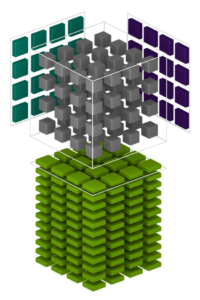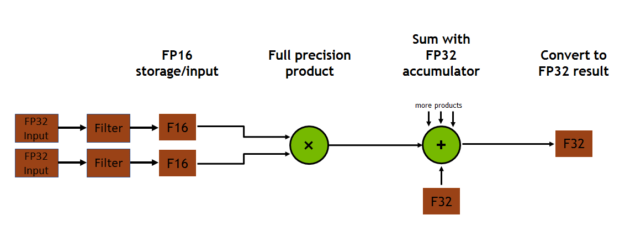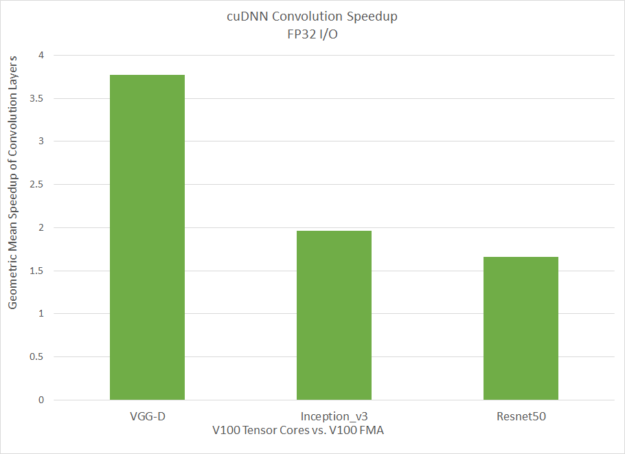# Tensor Ops Made Easier in cuDNNNeural network models have quickly taken advantage of NVIDIA Tensor Cores for deep learning since their introduction in the Tesla V100 GPU last year. For example, new performance records for ResNet50 training were announced recently with Tensor Core-based solutions. (See the NVIDIA developer post on new performance milestones for additional details).

NVIDIA’s cuDNN library enables CUDA programmers to optimize both recurrent neural networks and convolutional neural networks for GPU acceleration. We recently outlined easy ways for cuDNN users to take advantage of Tensor Cores for convolutions, complete with instructions and sample code. That article presented a few simple rules for cuDNN applications: FP16 data rules, tensor dimension rules, use of ALGO_1, etc.

Recent cuDNN versions now lift most of these constraints. The cuDNN 7.2 version lifted the FP16 data constraint, while cuDNN 7.3 removes the tensor dimension constraints (for packed NCHW tensor data). Let’s get right into the improvements.

## New: Use FP32 Data for Tensor Ops

The post on using Tensor Cores in CUDA discussed the use of FP16 input for tensor operations, as shown in figure 1. While tensor ops still consume FP16 data, the cuDNN API for convolutions now allows the user to choose to have FP32 input data converted to FP16. The output data of the convolution also are converted to FP32 if desired.Figure 1. FP32 data may now be used as input

The `CUDNN_TENSOR_OP_MATH_ALLOW_CONVERSION` enum value, new in cuDNN 7.2, enables the cuDNN applications programmer to choose to convert FP32 data for tensor op use. This enum value is passed to the `cudnnSetConvolutionMathType()` call, just as is the `CUDNN_TENSOR_OP_MATH` enum value. This code snippet shows how you might do this:

```// Set the math type to allow cuDNN to use Tensor Cores:
checkCudnnErr( cudnnSetConvolutionMathType(cudnnConvDesc, CUDNN_TENSOR_OP_MATH_ALLOW_CONVERSION) );
```

You can see the context in which code fragment is used in a later section.

### FP32 Data also for RNNs

Similar FP32 data conversions are now also enabled for RNNs. Simply pass the `CUDNN_TENSOR_OP_MATH_ALLOW_CONVERSION` enum value to the `cudnnSetRNNMatrixMathType()` call to allow FP32 data to be converted for use in your RNNs. Use this as follows:

```// Set the math type to allow cuDNN to use Tensor Cores:
checkCudnnErr( cudnnSetRNNMatrixMathType(cudnnRnnDesc, CUDNN_TENSOR_OP_MATH_ALLOW_CONVERSION) );
```

## New: NCHW Tensor Dimension Constraints Eliminated

Earlier versions of cuDNN required the channel dimension of all tensors  be a multiple of 8. That constraint no longer applies to packed NCHW data; cuDNN now automatically pads the tensors as needed.

This padding is automatic for packed NCHW data in both the `CUDNN_TENSOR_OP_MATH` and the `CUDNN_TENSOR_OP_MATH_ALLOW_CONVERSION` cases. The padding occurs with negligible loss of performance.

```// Set NCHW tensor dimensions, not necessarily as multiples of eight (only the input tensor is shown here):
int dimA[] = {1, 7, 32, 32};
int strideA[] = {7168, 1024, 32, 1};
```

The sample code in the section below demonstrates how you might use this.

## Sample Code

The logic to use tensor ops for FP32 data and any channel dimensions is similar to the logic used when writing for earlier versions of cuDNN. Only the dimensions and data types have changed (along with the use of `CUDNN_TENSOR_OP_MATH_ALLOW_CONVERSION)`:

```// Create a cuDNN handle:
checkCudnnErr(cudnnCreate(&handle_));

// Create your tensor descriptors:
checkCudnnErr( cudnnCreateTensorDescriptor( &cudnnIdesc ));
checkCudnnErr( cudnnCreateFilterDescriptor( &cudnnFdesc ));
checkCudnnErr( cudnnCreateTensorDescriptor( &cudnnOdesc ));
checkCudnnErr( cudnnCreateConvolutionDescriptor( &cudnnConvDesc ));

// Set NCHW tensor dimensions, not necessarily as multiples of eight (only the input tensor is shown here):
int dimA[] = {1, 7, 32, 32};
int strideA[] = {7168, 1024, 32, 1};

checkCudnnErr( cudnnSetTensorNdDescriptor(cudnnIdesc, CUDNN_DATA_FLOAT,
convDim+2, dimA, strideA) );

// Allocate and initialize tensors (again, only the input tensor is shown):
checkCudaErr( cudaMalloc((void**)&(devPtrI), (insize) * sizeof(devPtrI) ));
hostI = (T_ELEM*)calloc (insize, sizeof(hostI) );

initImage(hostI, insize);

checkCudaErr( cudaMemcpy(devPtrI, hostI, sizeof(hostI) * insize, cudaMemcpyHostToDevice));

// Set the compute data type (below as CUDNN_DATA_FLOAT):
checkCudnnErr( cudnnSetConvolutionNdDescriptor(cudnnConvDesc, convDim, padA, convstrideA, dilationA, CUDNN_CONVOLUTION, CUDNN_DATA_FLOAT) );

// Set the math type to allow cuDNN to use Tensor Cores:
checkCudnnErr( cudnnSetConvolutionMathType(cudnnConvDesc, CUDNN_TENSOR_OP_MATH_ALLOW_CONVERSION) );

// Choose a supported algorithm:
cudnnConvolutionFwdAlgo_t algo = CUDNN_CONVOLUTION_FWD_ALGO_IMPLICIT_PRECOMP_GEMM;

// Allocate your workspace:
checkCudnnErr( cudnnGetConvolutionForwardWorkspaceSize(handle_, cudnnIdesc,
cudnnFdesc, cudnnConvDesc,
cudnnOdesc, algo, &workSpaceSize) );

if (workSpaceSize > 0) {
cudaMalloc(&workSpace, workSpaceSize);
}

// Invoke the convolution:
checkCudnnErr( cudnnConvolutionForward(handle_, (void*)(&alpha), cudnnIdesc, devPtrI,
cudnnFdesc, devPtrF, cudnnConvDesc, algo,
workSpace, workSpaceSize, (void*)(&beta),
cudnnOdesc, devPtrO) );
```

## FP32 Performance

Figure 2 shows the comparative performance of convolutions when using Tensor Cores for FP32 tensor data. The chart compares V100 tensor ops versus V100 FMA ops, so the gains are not quite as dramatic as earlier charts comparing V100 performance versus P100 FMA. Tensor ops used with FP32 input still represent significant gains over using FMA ops, nonetheless.Figure 2. Performance comparison of convolution on Tesla V100 (Volta) with Tensor Cores versus Tesla V100 (Volta). The comparison is between the geometric means of run times of the convolution layers from each neural network. Both cases use FP32 input/output data and FP32 computation; one uses Tensor Cores, while the other uses FP32 fused-multiply add (FMA).

## Remaining Constraints

While the major constraints for using tensor ops in cuDNN have been lifted, some minor constraints still remain. One limitation is the use of ALGO_1 (`IMPLICIT_PRECOMP_GEMM` for forward). No other convolution ALGOs in cuDNN make use of tensor ops yet.

Another minor restriction is the size of the convolution filter, specifically the spatial dimensions (r and s). However, the FFT algorithms for convolution are very well suited for use cases with large filter dimensions. Just switch your convolutions to use FFT algorithms well before the tensor op filter limits are exceeded for maximum performance.

## Get Started with Tensor Cores in cuDNN Today

You can download the latest version of cuDNN here and get started using Tensor Cores today. See how Tensor Cores can supercharge your cuDNN applications. Read the latest Release Notes for a detailed list of new features and enhancements.